﻿

### Canonical decompositions induced by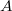$A$-contractions

#### Abstract

The classical Nagy-Foia\c s-Langer decomposition of an ordinary contraction is generalized in the context of the operators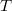$T$ on a complex Hilbert space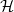$\mathcal{H}$ which, relative to a positive operator$A$ on$\mathcal{H}$, satisfy the inequality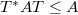$T^*AT \le A$. As a consequence, a version of the classical von Neumann-Wold decomposition for isometries is derived in this context. Also one shows that, if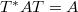$T^*AT=A$ and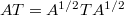$AT=A^{1/2}TA^{1/2}$, then the decomposition of$\mathcal{H}$ in normal part and pure part relative to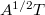$A^{1/2}T$ is just a von Neumann-Wold type decomposition for$A^{1/2}T$, which can be completely described.  As applications, some facts on the quasi-isometries recently studied in , , are obtained.

DOI Code: 10.1285/i15900932v28n2p187

Keywords: A-contraction; A-isometry; quasi-isometry; von Neumann-Wold decomposition

Classification: 47A15; 47A63; 47B20

Full Text: PDF